MathScore EduFighter is one of the best math games on the Internet today. You can start playing for free!

## Identifying Angles - Sample Math Practice Problems

The math problems below can be generated by MathScore.com, a math practice program for schools and individual families. References to complexity and mode refer to the overall difficulty of the problems as they appear in the main program. In the main program, all problems are automatically graded and the difficulty adapts dynamically based on performance. Answers to these sample questions appear at the bottom of the page. This page does not grade your responses.

See some of our other supported math practice problems.

### Complexity=5, Mode=twoline

Classify the following angles that are labeled.

 1.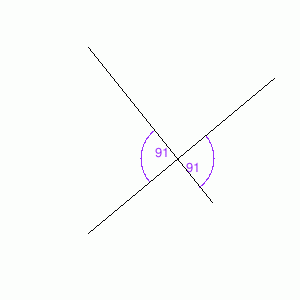Vertical Angles        Adjacent Angles        Complementary Angles        Supplementary Angles        Corresponding Angles        None of the above 2.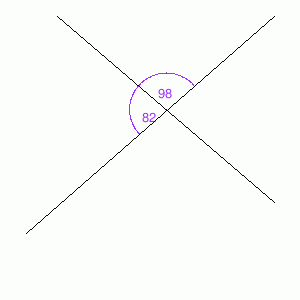Vertical Angles        Adjacent Angles        Complementary Angles        Supplementary Angles        Corresponding Angles        None of the above

### Complexity=8, Mode=threeline

Classify the following angles that are labeled.

 1.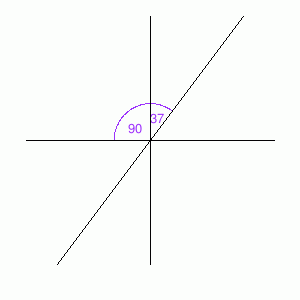Vertical Angles        Adjacent Angles        Complementary Angles        Supplementary Angles        Corresponding Angles        None of the above 2.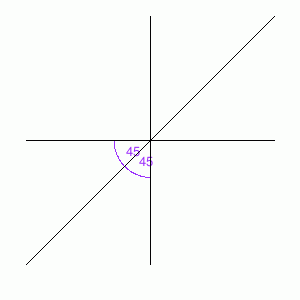Vertical Angles        Adjacent Angles        Complementary Angles        Supplementary Angles        Corresponding Angles        None of the above

### Complexity=10, Mode=fourline

Classify the following angles that are labeled.

 1.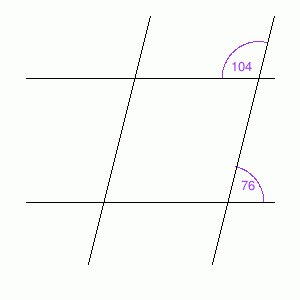Vertical Angles        Adjacent Angles        Complementary Angles        Supplementary Angles        Corresponding Angles        None of the above 2.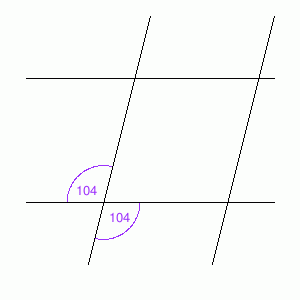Vertical Angles        Adjacent Angles        Complementary Angles        Supplementary Angles        Corresponding Angles        None of the above

### Complexity=20

Classify the following angles that are labeled.

 1.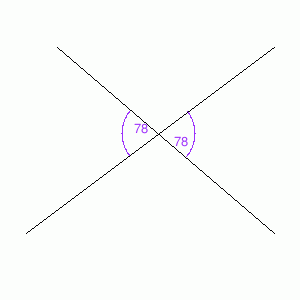Vertical Angles        Adjacent Angles        Complementary Angles        Supplementary Angles        Corresponding Angles        None of the above 2.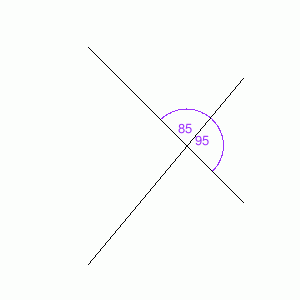Vertical Angles        Adjacent Angles        Complementary Angles        Supplementary Angles        Corresponding Angles        None of the above

### Complexity=5, Mode=twoline

Classify the following angles that are labeled.

1Vertical Angles
Complementary Angles
Supplementary Angles
Corresponding Angles
None of the above
Solution
Since the two angles are formed by the intersection of the same two lines, they are vertical angles.
2Vertical Angles
Complementary Angles
Supplementary Angles
Corresponding Angles
None of the above
Solution
Since the two angles share a common side, the two angles are adjacent angles.
Since the two angles add up to 180 degrees, they are supplementary angles.

### Complexity=8, Mode=threeline

Classify the following angles that are labeled.

1Vertical Angles
Complementary Angles
Supplementary Angles
Corresponding Angles
None of the above
Solution
Since the two angles share a common side, the two angles are adjacent angles.
2Vertical Angles
Complementary Angles
Supplementary Angles
Corresponding Angles
None of the above
Solution
Since the two angles share a common side, the two angles are adjacent angles.
Since the two angles add up to 90 degrees, they are complementary angles.

### Complexity=10, Mode=fourline

Classify the following angles that are labeled.

1Vertical Angles
Complementary Angles
Supplementary Angles
Corresponding Angles
None of the above
Solution
Since the two angles add up to 180 degrees, they are supplementary angles.
2Vertical Angles
Complementary Angles
Supplementary Angles
Corresponding Angles
None of the above
Solution
Since the two angles are formed by the intersection of the same two lines, they are vertical angles.

### Complexity=20

Classify the following angles that are labeled.

1Vertical Angles
Complementary Angles
Supplementary Angles
Corresponding Angles
None of the above
Solution
Since the two angles are formed by the intersection of the same two lines, they are vertical angles.
2Vertical Angles